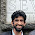Jan 31, 2011

Comparison without relational operators

Source: Quant interview at Religare Technova

Problem: Write a C program to compare two integers without using relational operators (== != < <= > >=)

1.int d = a - b;
if(d)
{
// a is not equal to b

if( d - abs(d) )
{
// d was negative => b > a
}
else
{
// d was positive => a > b
}
}
else
{
// a == b :)
}

1.In the implementation of abs(), a relational operator is used to determine whether the number passed is less than or greater than zero.

2.say 2 nos are a and b in their n-bit representations. subtract b from a by taking 2's complement of b and adding it to a, multiply it by 100...(n-1 0s). then right-shift the no n-1 times. call it c. multiply c by b. then complement c, multiply it by a. now max = b*c+a*(complement of c)

3.Will this work?

int compare(int64_t a, int64_t b)
{
return ((uint64_t) (a - b)) >> 63;
}

4.Sorry but i couldn't make it simpler..

#include
#include
int maximum(int x,int y)
{
return(x+y+sqrt(-2*y*x +x*x + y*y))/2;
}

int main()
{
int a,b,c;
scanf("%d%d",&a,&b);
c=maximum(a,b);
printf("\n%d",c);
}

5.int main () {

int a,b;
printf ( "enter the two values u like to compare\n");
scanf (" %d %d",&a,&b);

if (!(a ^ b))
printf ("both are equal\n);
else
printf ("both are not equal\n");
}

6.int ret_max(int a, int b){
int c=a,d=b;
while (c&d){
c&=(c-1);
c&=(d-1);
}
if (c)
return a;
else // also takes care of equality
return b;
}

7.//to check if no's are equal
if(!(a^b))
print(equal)
else if (a/b)
print(b greater than a)
else
print(a greater than b)

Fraction Brainteaser

Source: Sent to me by Gaurav Sinha Problem: Siddhant writes a Maths test and correctly answers 5 out of 6 Arithmetic questions and 20...